# Divisibility - math problems

#### Number of problems found: 226

• 5 digitsYou have the following digits: 9, 8, 0, 1, 5. Write the smallest even five-digit number if one digit is repeated three times and the other digits are not repeated. The digit sum of the number is: a) 9 b) 6 c) 8 d) 23
• What is 16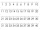What is the sum of three consecutive even integers such that six more than twice the second is 2/3 of the first increased by 3/2 of the third?
• AND-NOT-ANDIf P is the set of multiples of 2, Q is the set of multiples of 3, and R is the set of multiples of 7, which of the following integers will be in P and Q but not in R? A=−54 B=−50 C=42 D=100 E=252
• Check divisibility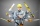Put a check under each column to identify whether each number is divisible by 2, 5, 10, 3, 6 or 9. @TB@ 54180#1 624 2700 5605 568 @TE@
• Dividing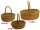When dividing the tangerines into packages of 8 or 10, one always remained. How many were there if there were more than 250 and less than 300?
• Lcm = 22 + gcdThe least common multiple of two numbers is 22 more than their greatest common divisor. Find these numbers.
• Most divisorsFrom the natural numbers from 1 to 100, find the one that has the most divisors.
• The largest divisorThe largest number which divides 60 and 75 leaving remainders 8 and 10 respectively is:
• Eights of butter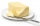How many eights of butter (1/8 of kg = 125 g) can be stored in a box with dimensions of 4 dm, 2 dm, 1.8 dm, if the eighth of butter has dimensions of 8 cm, 5 cm, 3 cm?
• What are 3What are the two digits which when inserted in the blank spaces will make 234 _ _ divisible by 8?
• Four multiplesFour multiples of 6, he writes the following 12  24  56  72, which is correct?
• The dice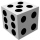Find the probability that a number divisible by two or three will fall when the dice are rolled.
• Three-digit numbersHow many are all three-digit numbers that are made up of digits 0,2,5,7 and are divisible by 9 if the digits can be repeated?
• We rollWe roll two dice A. - what is the probability that the sum of the falling numbers is at most 4 B. - is at least 10 C. - is divisible by 5?
• Five-digit numberAnna thinks of a five-digit number that is not divisible by three or four. If he increments each digit by one, it gets a five-digit number that is divisible by three. If he reduces each digit by one, he gets a five-digit number divisible by four. If it sw
• Even/odd numbersWhat is the product of 0.97 and the next odd decimal number?
• TogetherThe three friends divided the balls in a ratio of 6: 5: 4. Some two of them got a total of 126 balls. How many balls were there together?
• Find unknown numberWhat is the number between 50 and 55 that is divisible by 2,3,6,9?
• CalculateCalculate the sum of all three-digit natural numbers divisible by five.
• Coloured numbersMussel wrote four different natural numbers with coloured markers: red, blue, green and yellow. When the red number divides by blue, it gets the green number as an incomplete proportion, and yellow represents the remainder after this division. When it div

Do you have an exciting math question or word problem that you can't solve? Ask a question or post a math problem, and we can try to solve it.

We will send a solution to your e-mail address. Solved examples are also published here. Please enter the e-mail correctly and check whether you don't have a full mailbox.